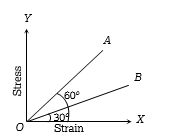The stress versus strain graphs for wires of two materials A and B are as shown in the figure. If ${Y}_{A}$ and ${Y}_{B}$ are the Young ‘s modulii of the materials, then(a) ${Y}_{B}=2{Y}_{A}$

(b) ${Y}_{A}={Y}_{B}$

(c) ${Y}_{B}=3{Y}_{A}$

(d) ${Y}_{A}=3{Y}_{B}$

Concept Questions :-

Stress-strain curve
High Yielding Test Series + Question Bank - NEET 2020

Difficulty Level:

The Young's modulus of a wire is Y.  If the energy per unit volume is E, then the strain will be

(a) $\sqrt{\frac{2E}{Y}}$                                         (b) $\sqrt{2EY}$

(c) $EY$                                              (d) $\frac{E}{Y}$

Concept Questions :-

Stress-strain
High Yielding Test Series + Question Bank - NEET 2020

Difficulty Level:

If a spring extends by x on loading, then the energy stored by the spring is (if T is tension in the spring and k is spring constant)

(a) $\frac{{T}^{2}}{2x}$                                      (b) $\frac{{T}^{2}}{2k}$

(c) $\frac{2x}{{T}^{2}}$                                      (d) $\frac{2{T}^{2}}{k}$

Concept Questions :-

Stress-strain
High Yielding Test Series + Question Bank - NEET 2020

Difficulty Level:

When a force is applied on a wire of uniform cross-sectional area $3×{10}^{-6}{m}^{2}$ and length 4m, the increase in length is 1 mm. Energy stored in it will be $\left(Y=2×{10}^{11}N/{m}^{2}\right)$

1. 6250 J                               2. 0.177 J

3. 0.075 J                              4. 0.150 J

Concept Questions :-

Stress-strain
High Yielding Test Series + Question Bank - NEET 2020

Difficulty Level:

A wire of length L and cross-sectional area A is made of a material of Young's modulus Y. It is stretched by an amount x. The work done is -

(a) $\frac{YxA}{2L}$                             (b) $\frac{Y{x}^{2}A}{L}$

(c) $\frac{Y{x}^{2}A}{2L}$                           (d) $\frac{2Y{x}^{2}A}{L}$

Concept Questions :-

Hooke's law

Difficulty Level:

A stretched rubber has

(a) Increased kinetic energy

(b) Increased potential energy

(c) Decreased kinetic energy

(d) Decreased potential energy

Concept Questions :-

Elasticity

Difficulty Level:

When load of 5kg is hung on a wire then extension of 3m takes place, then work done will be

(a) 75 joule                             (b) 60 joule

(c) 50 joule                             (d) 100 joule

Concept Questions :-

Stress-strain
High Yielding Test Series + Question Bank - NEET 2020

Difficulty Level:

The work per unit volume to stretch the length by 1% of a wire with constant cross sectional area   will be. $\left[Y=9×{10}^{11}N/{m}^{2}\right]$

(a)                           (b) $4.5×{10}^{7}J$

(c) $9×{10}^{7}J$                            (d)

Concept Questions :-

Stress-strain
High Yielding Test Series + Question Bank - NEET 2020

Difficulty Level:

The ratio of Young's modulus of the material of two wires is 2 : 3. If the same stress is applied on both, then the ratio of elastic energy per unit volume will be-

(a) 3 : 2                                   (b) 2 : 3

(c) 3 : 4                                    (d) 4 : 3

Concept Questions :-

Stress-strain
High Yielding Test Series + Question Bank - NEET 2020

Difficulty Level:

A wire is suspended by one end. At the other end a weight equivalent to 20 N force is applied. If the increase in length is 1.0 mm, the increase in energy of the wire will be

(a) 0.01 J                                (b) 0.02 J

(c) 0.04 J                                (d) 1.00 J

Concept Questions :-

Stress-strain